# RD Sharma Solutions for Class 12 Maths Chapter 3 Binary Operations

## RD Sharma Solutions Class 12 Maths Chapter 3 – Free PDF Download Updated for (2021-22)

The RD Sharma Solutions for Class 12 Maths Chapter 3 – Binary Operations solutions are available here in PDF form. Students who are unable to solve exercise-wise problems of Chapter 3 can download the solutions PDF of RD Sharma Solutions. The RD Sharma Solutions are created by expert subject tutors according to the latest CBSE guidelines and marking schemes. Further, these solutions are presented in a step-wise manner for better learning of concepts.

The RD Sharma Solutions for Class 12 are explained in a simple and precise way as per the CBSE board. Students who wish to perform well in the Class 12 exam can use PDF as a major study material to boost their exam preparation. In order to gain a better knowledge of the concepts covered here, students can access RD Sharma Solutions for Class 12 Chapter 3 Binary Operations PDF, from the links which are provided here.

## Download the PDF of RD Sharma Solutions For Class 12 Maths Chapter 3 Binary Operations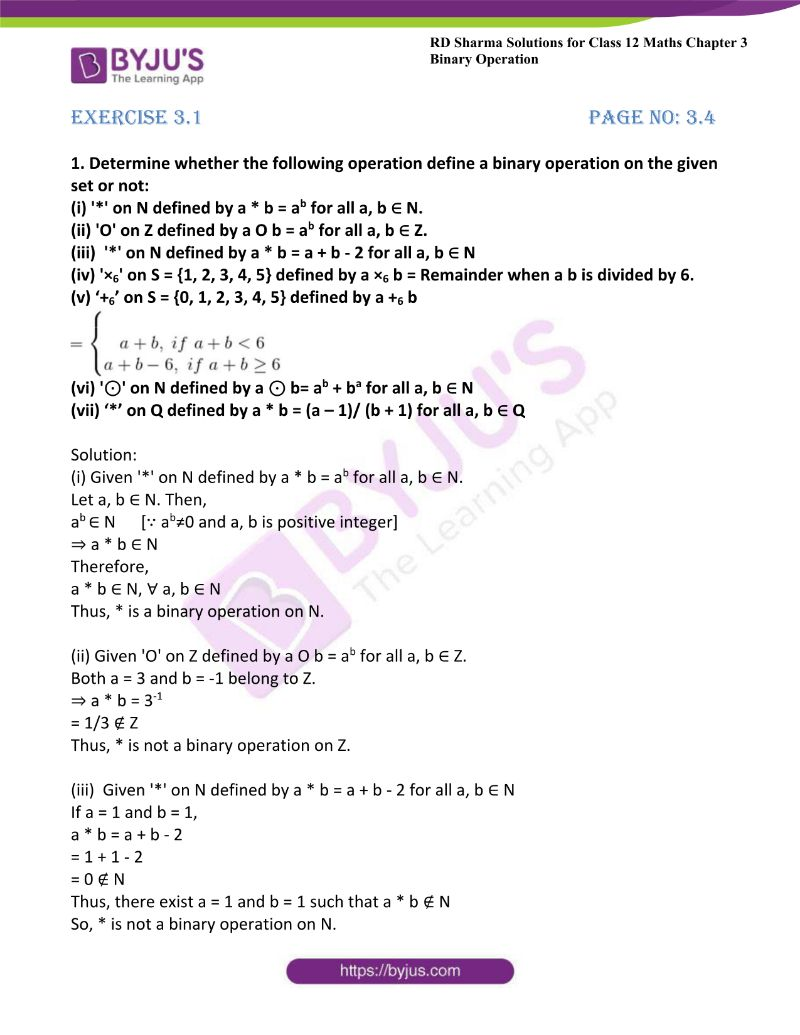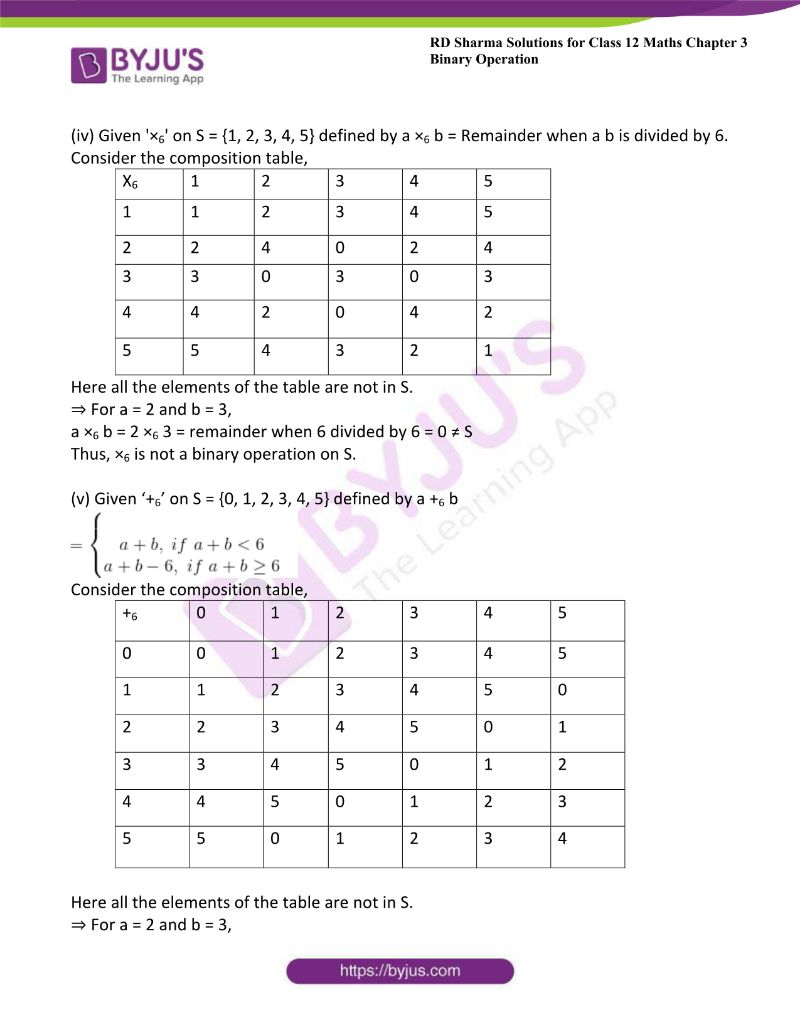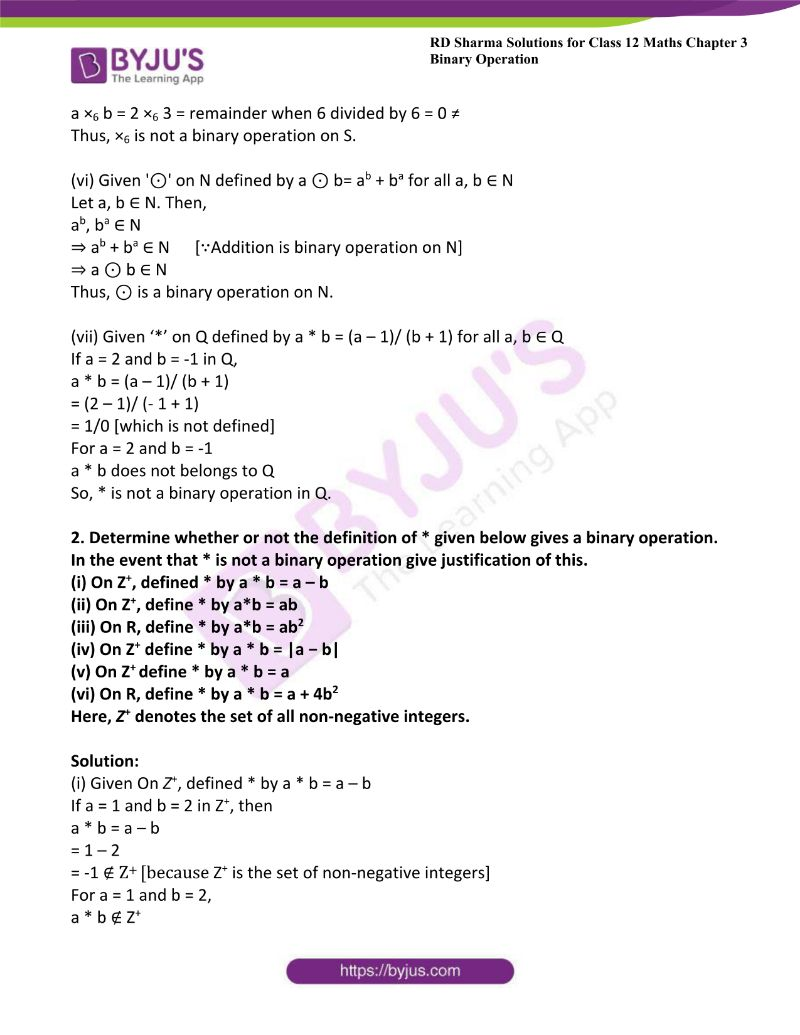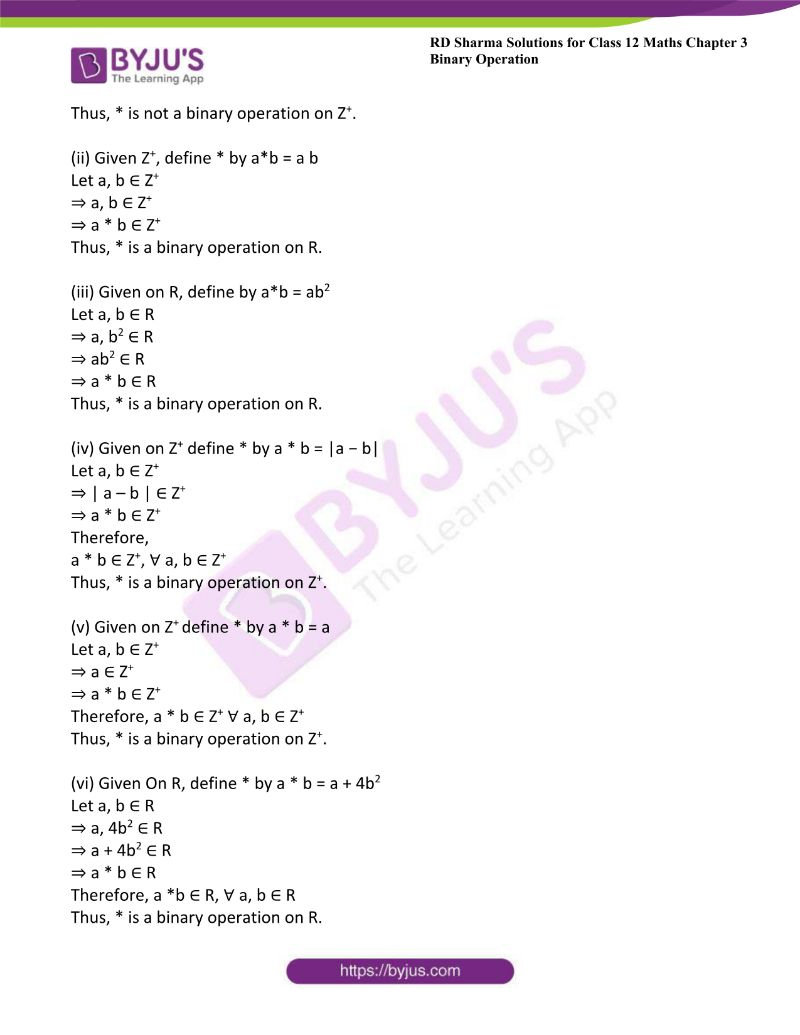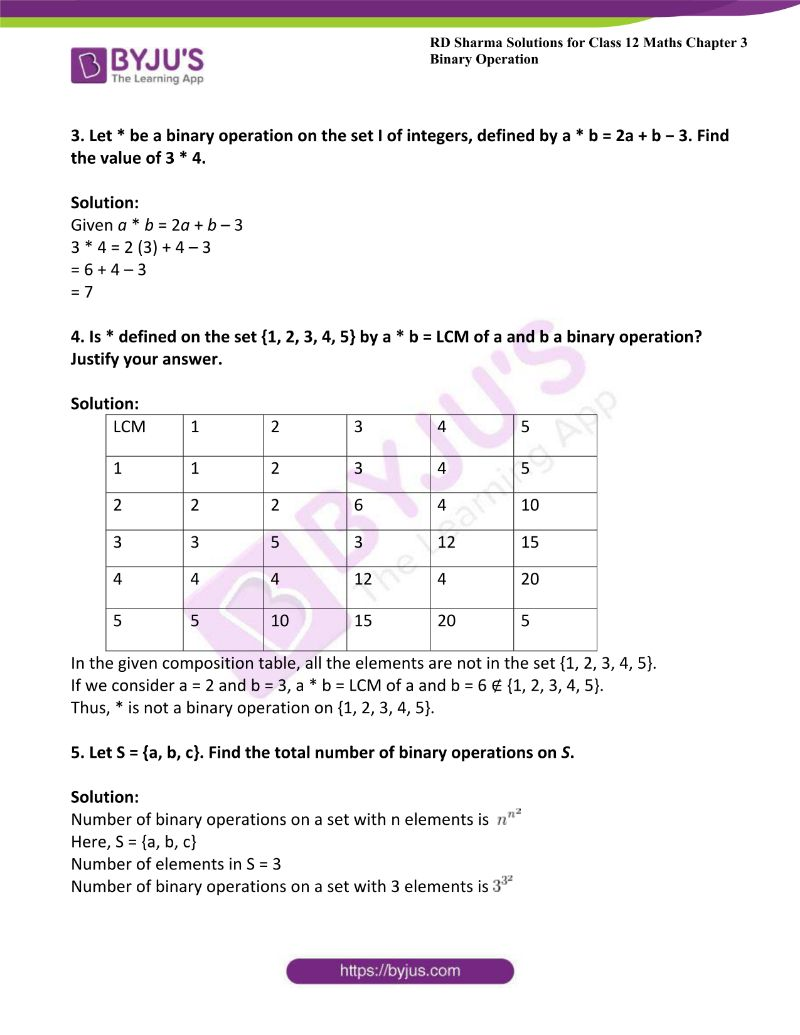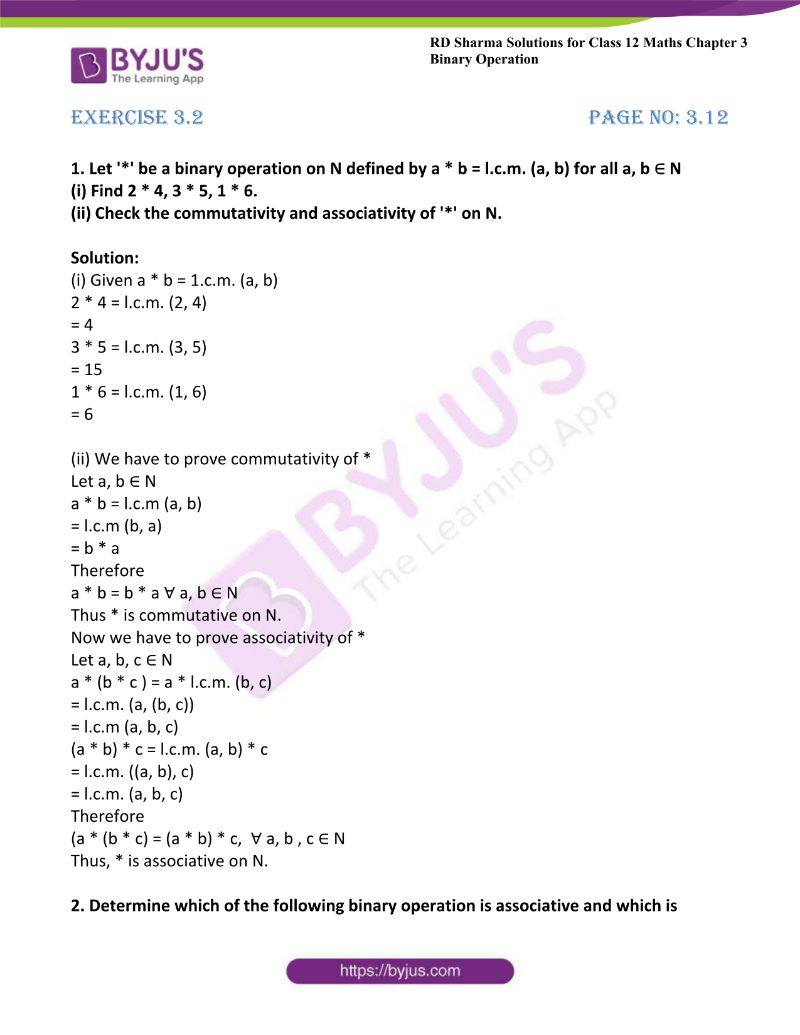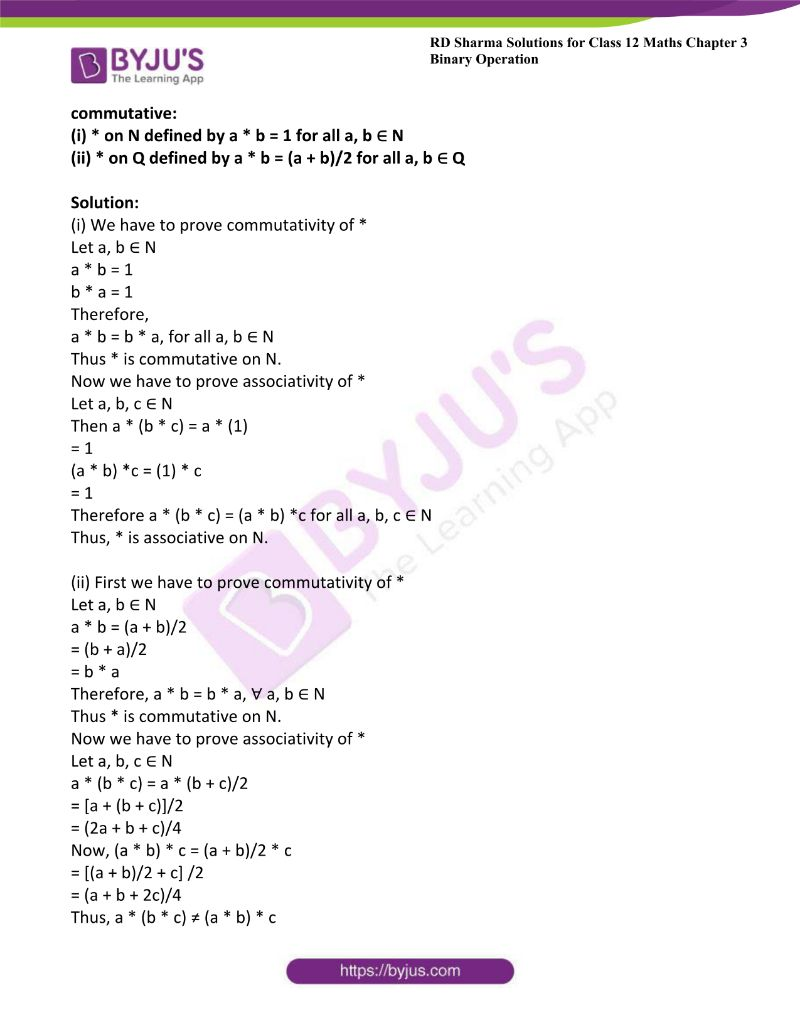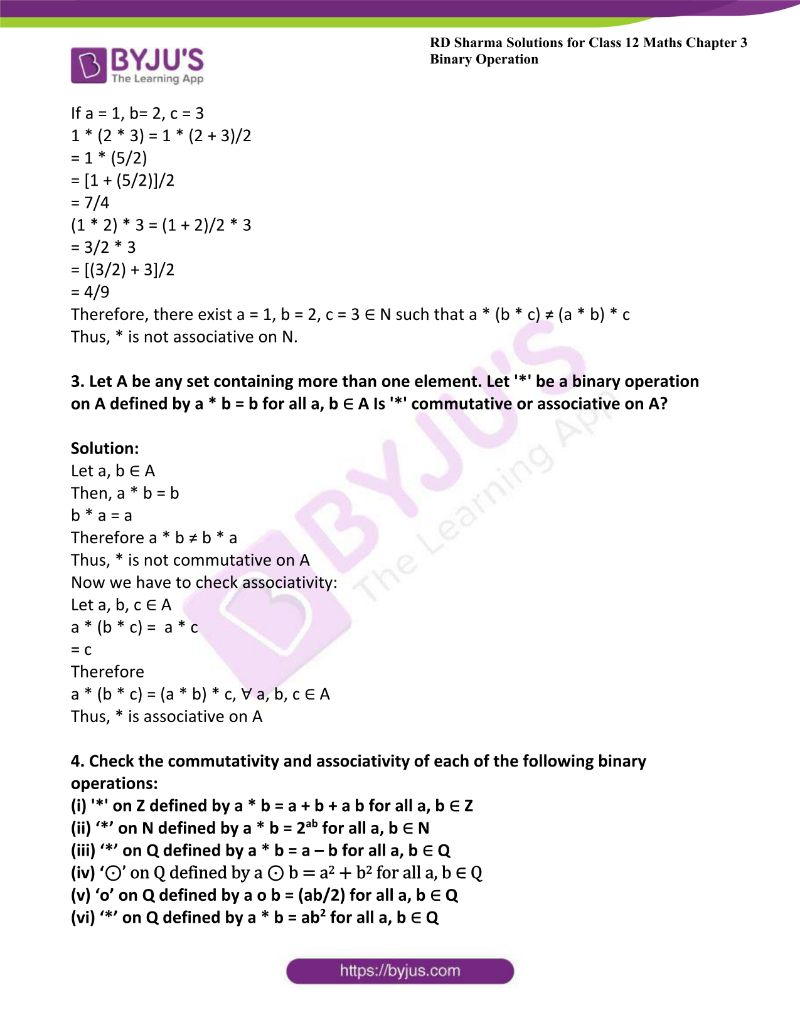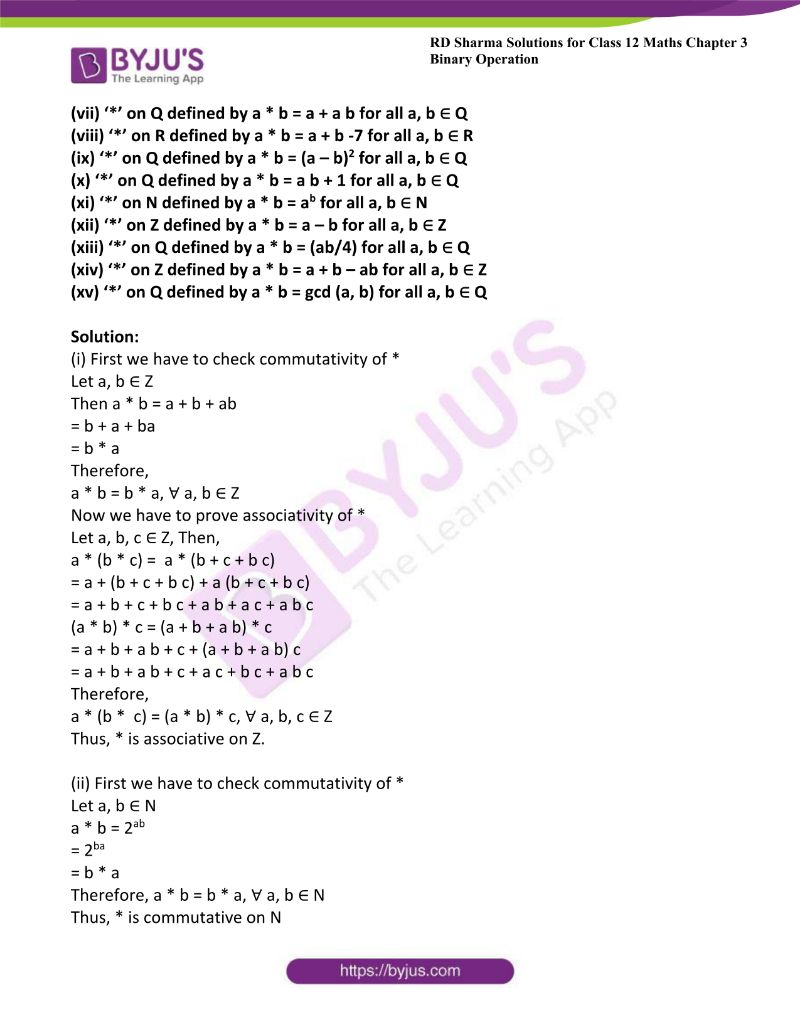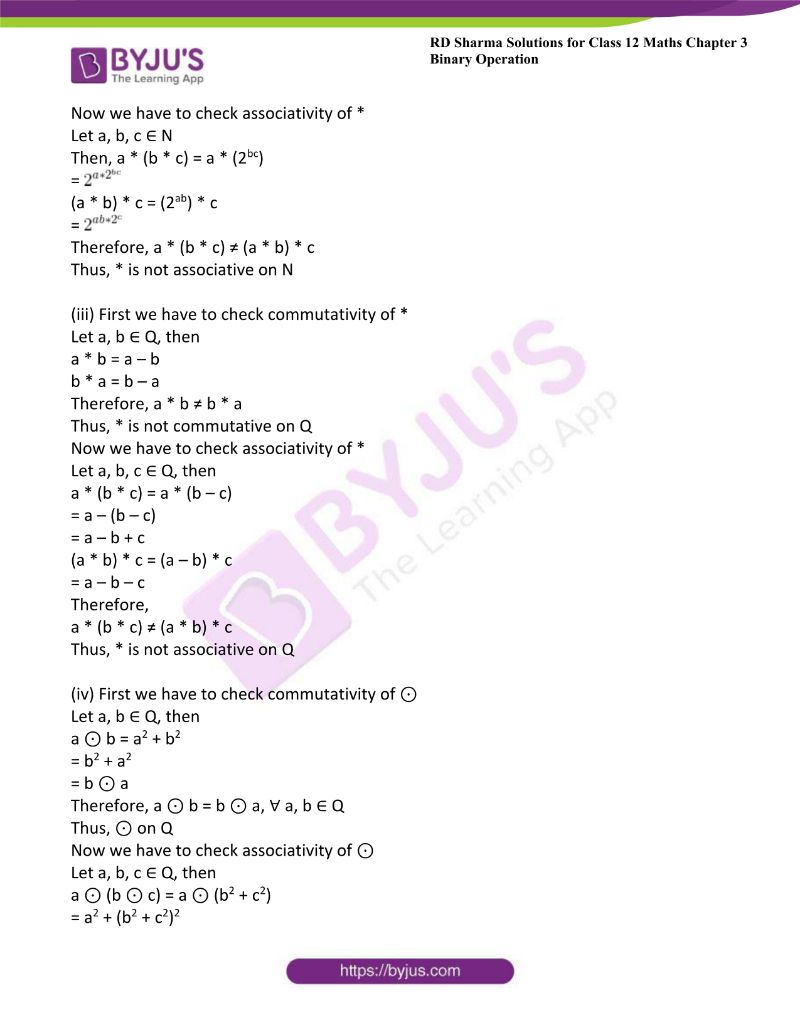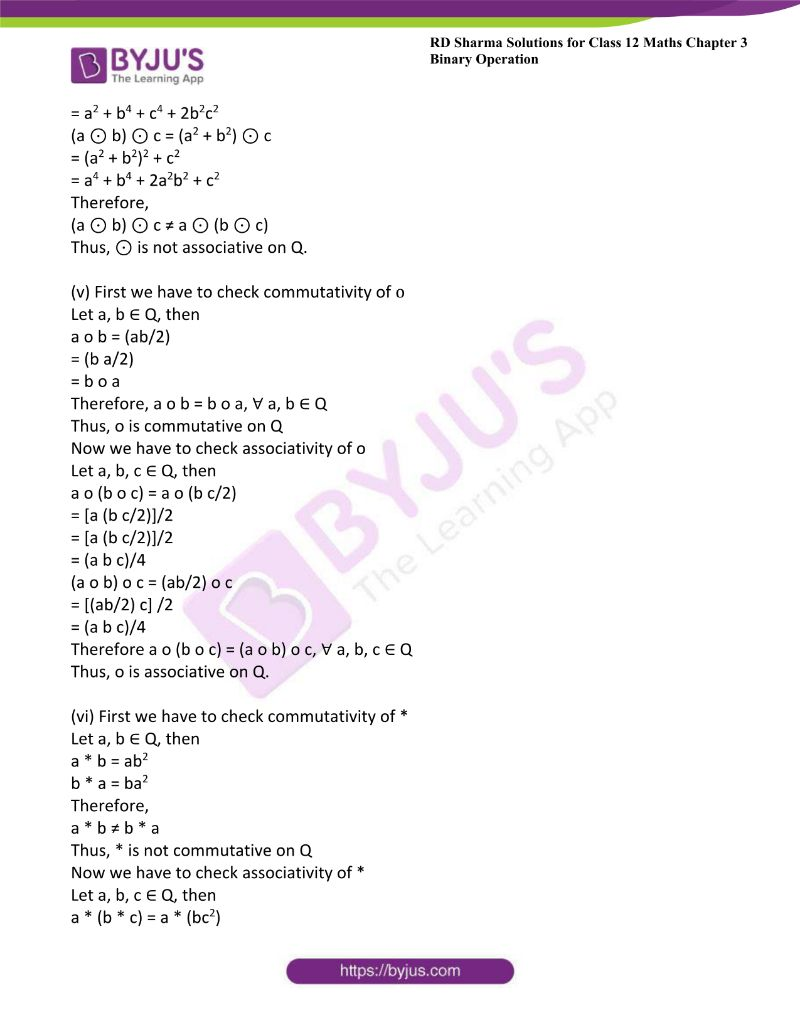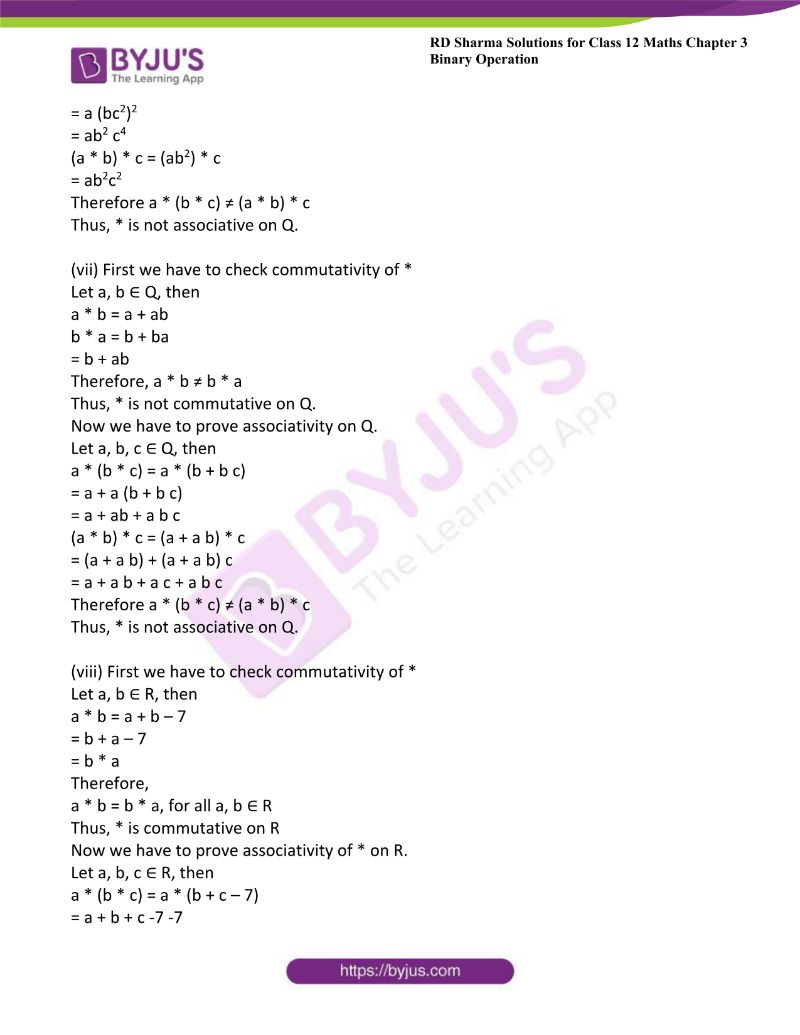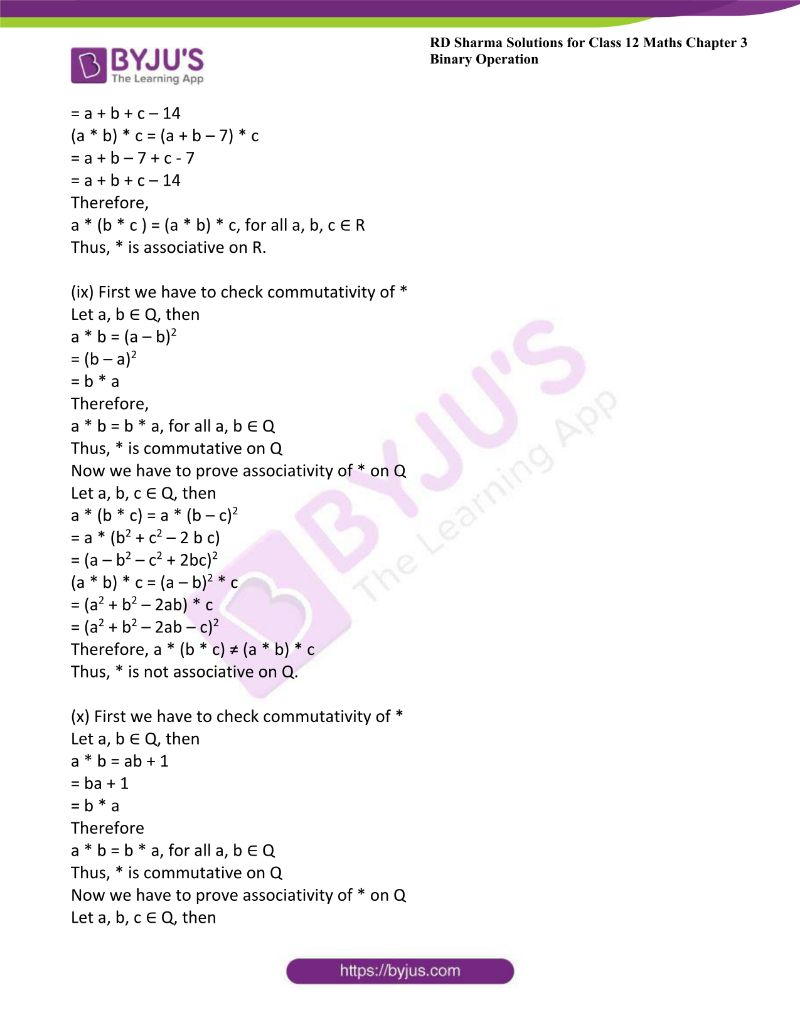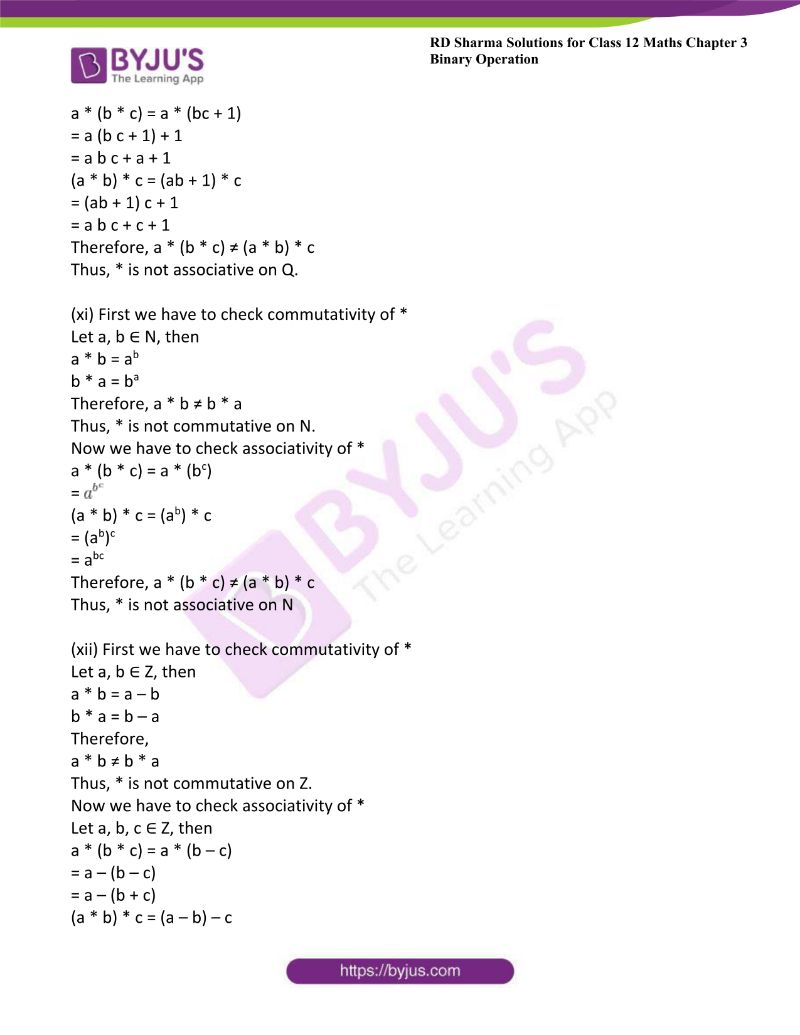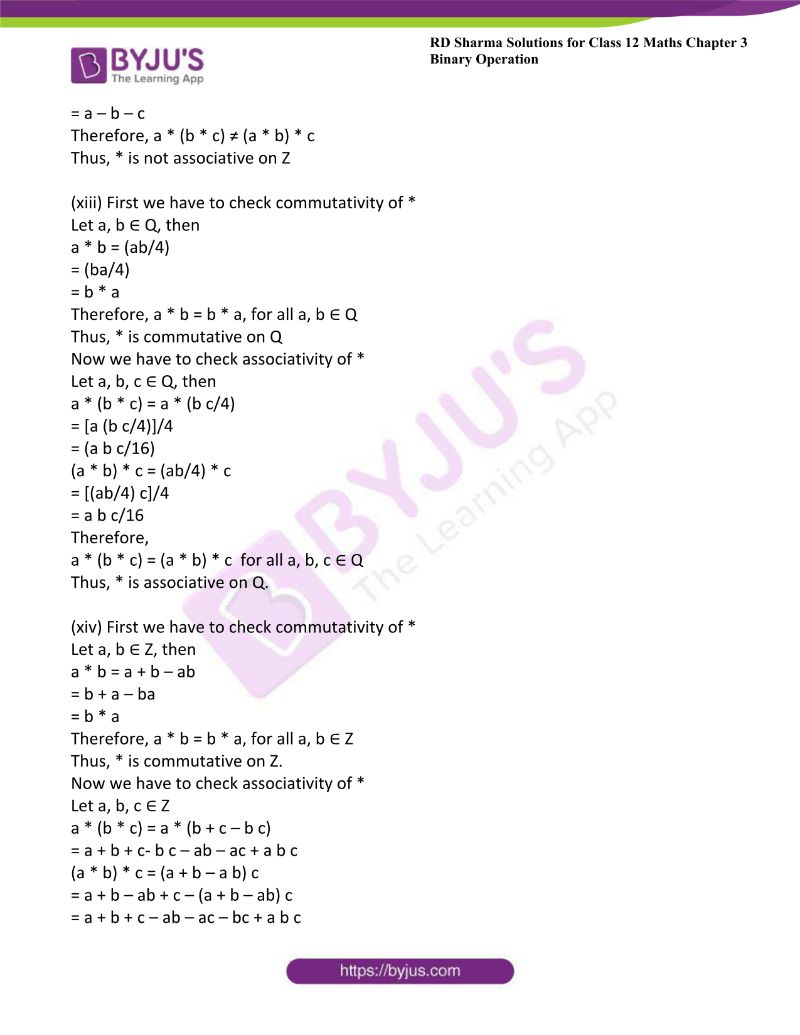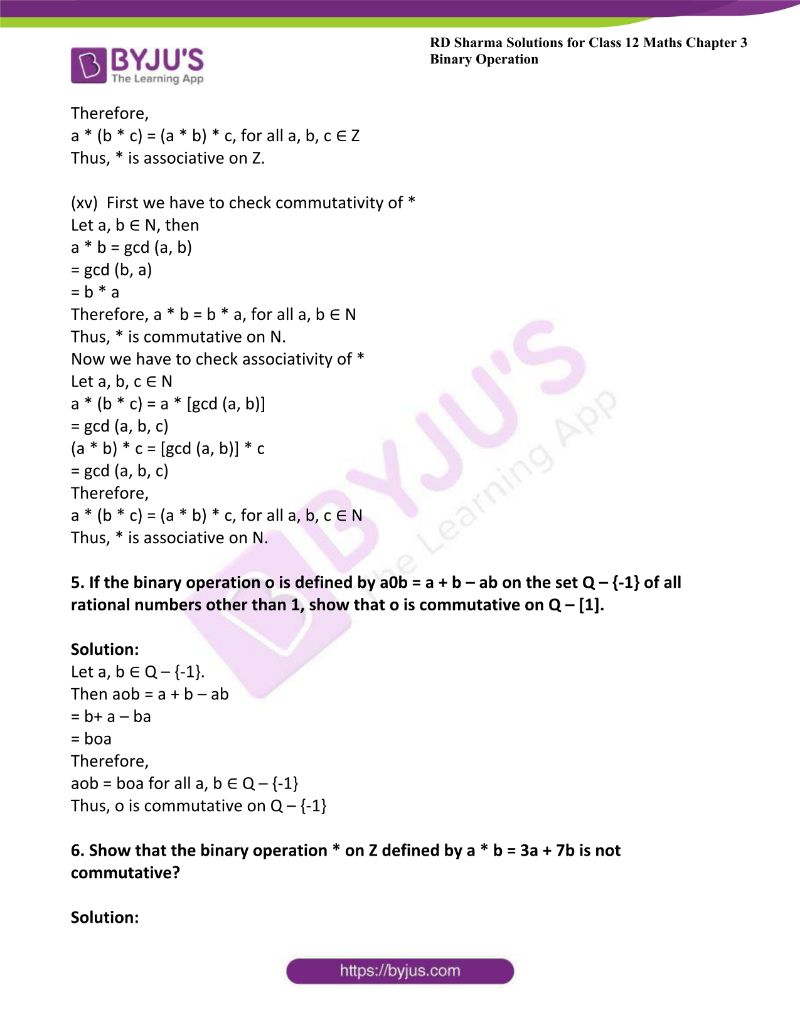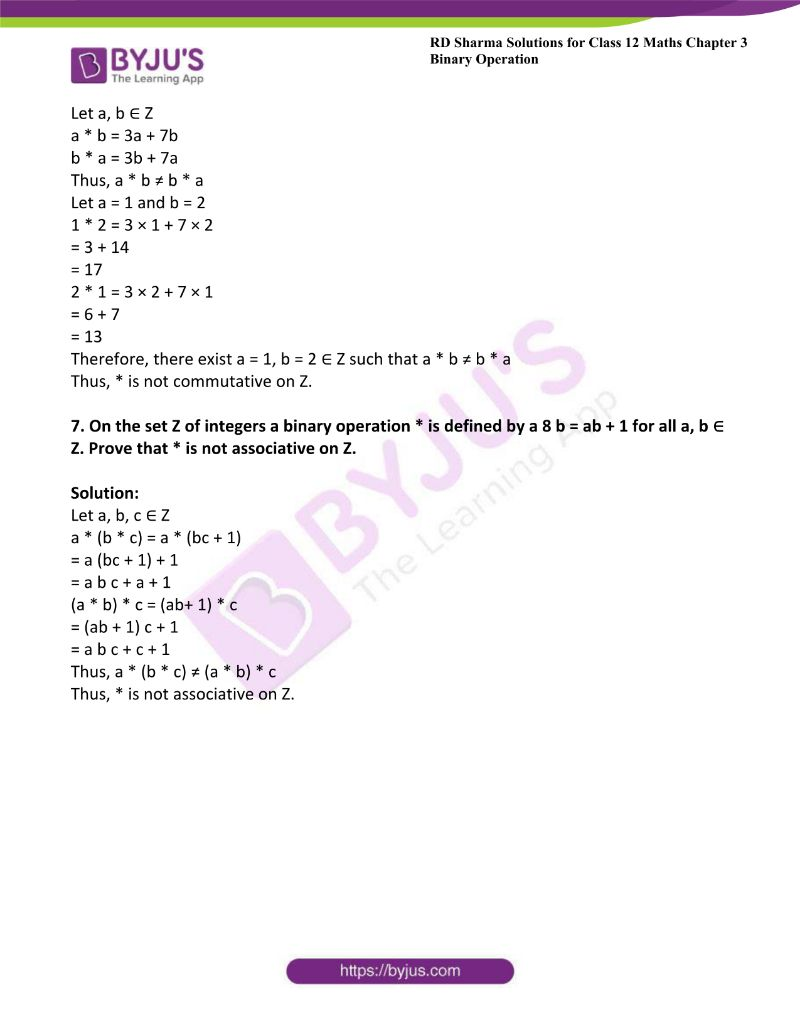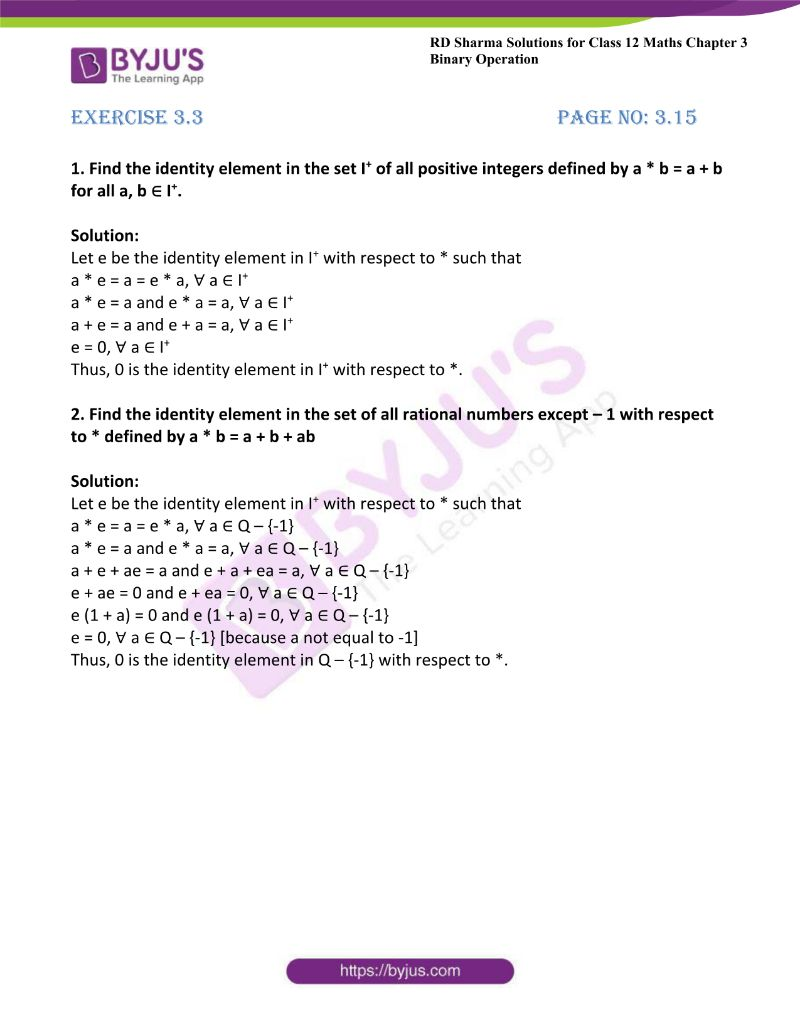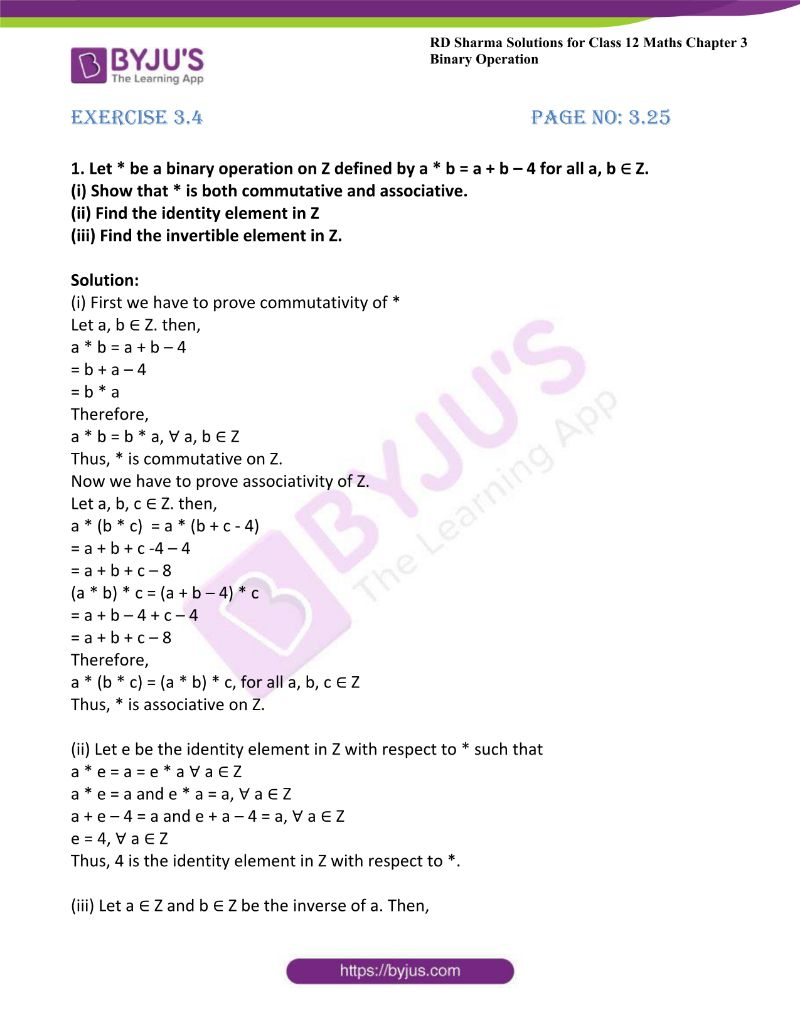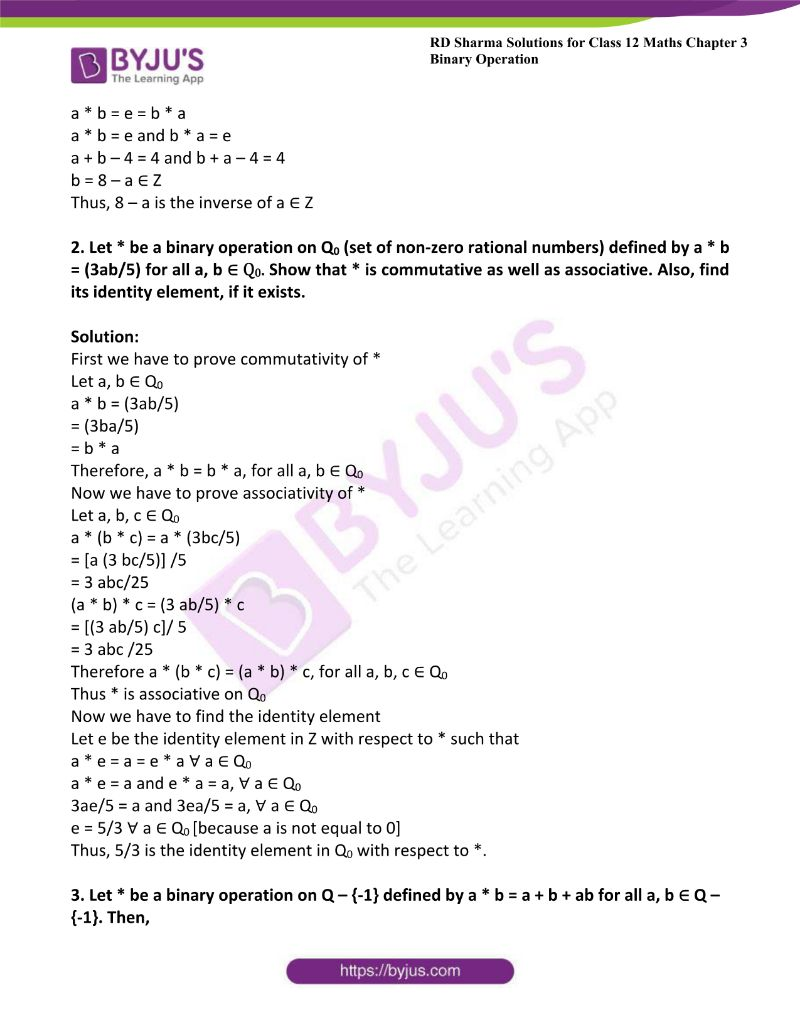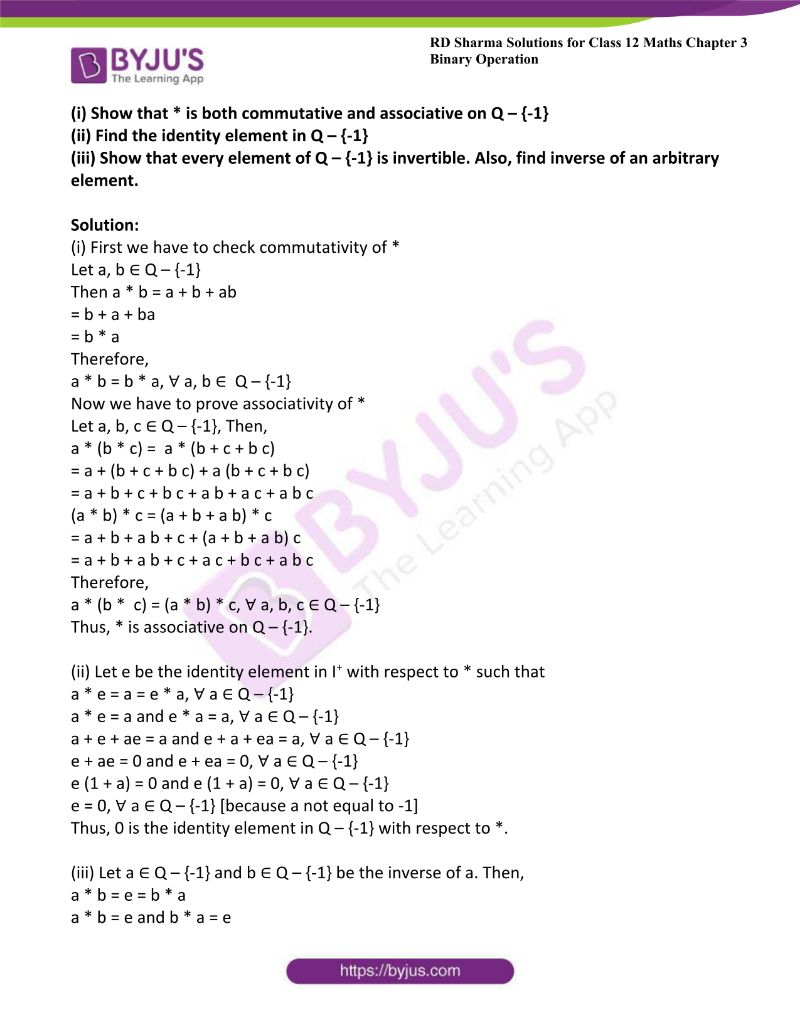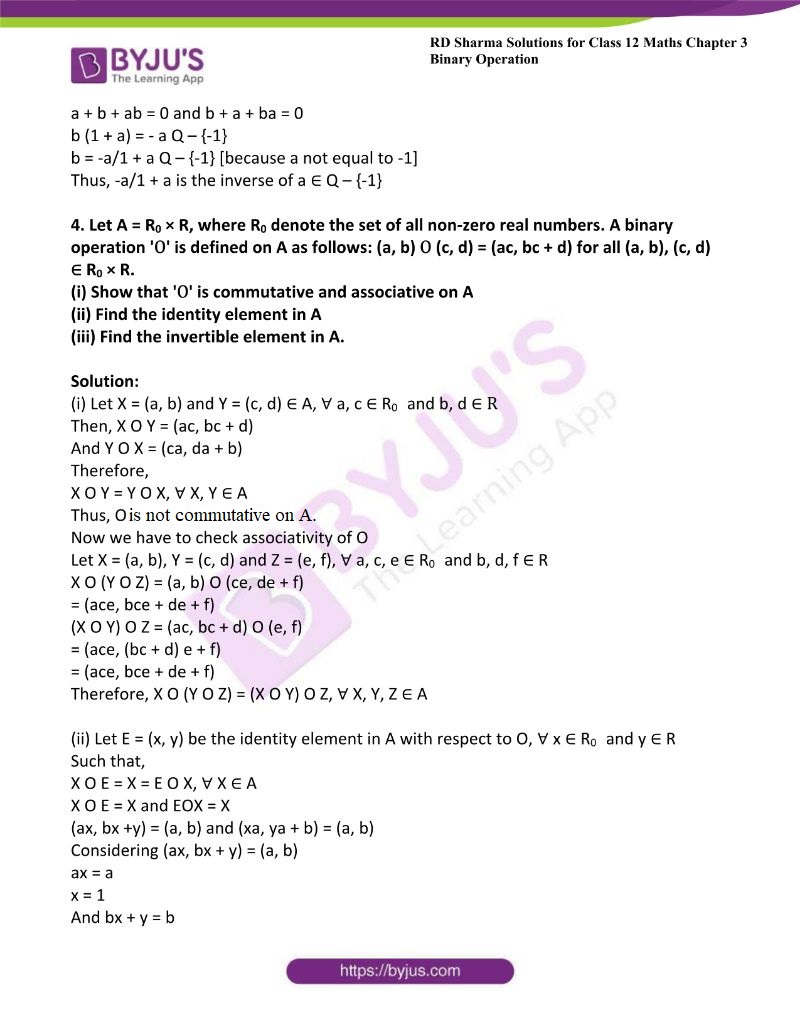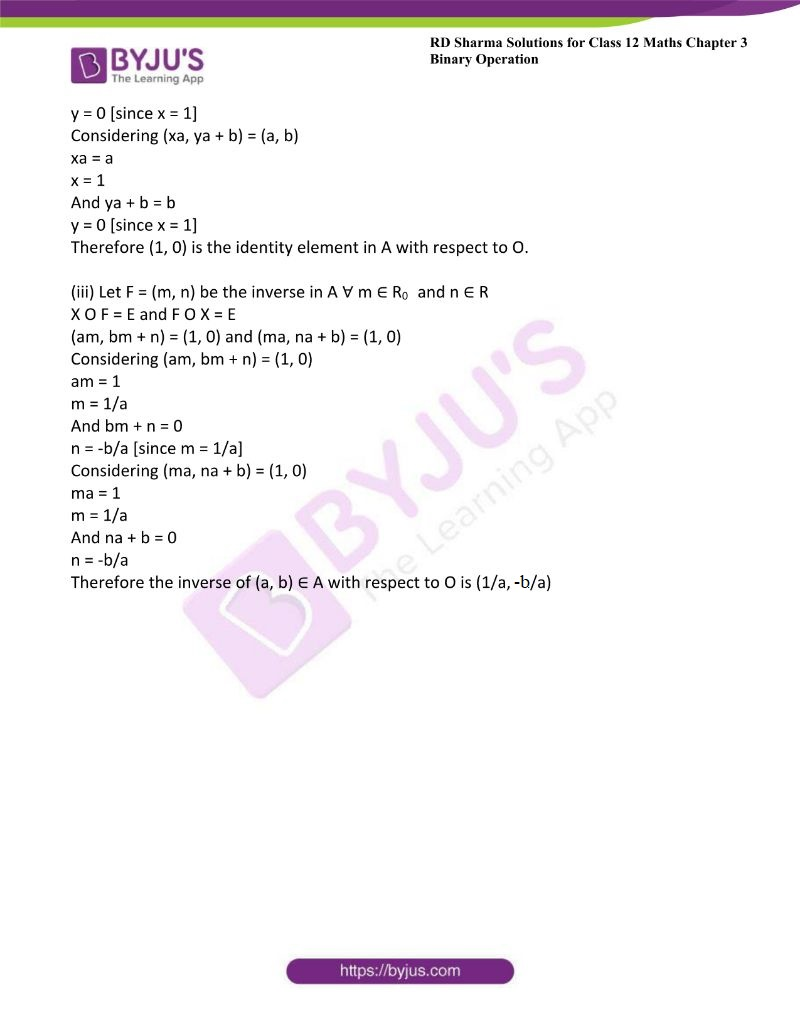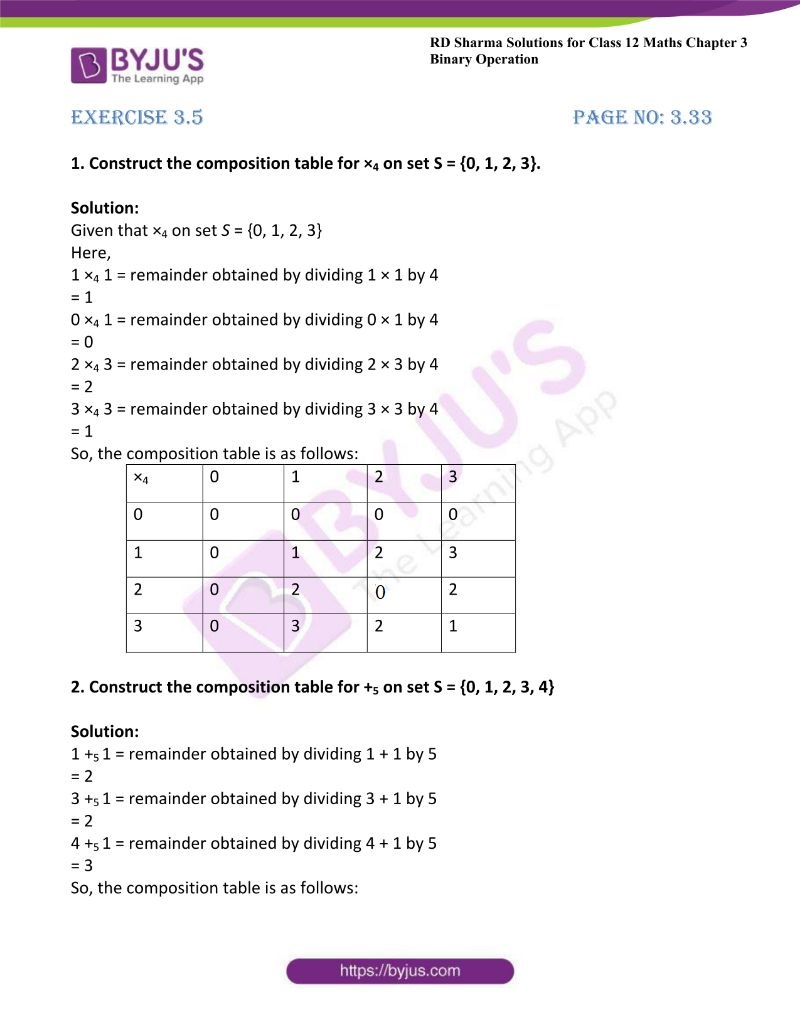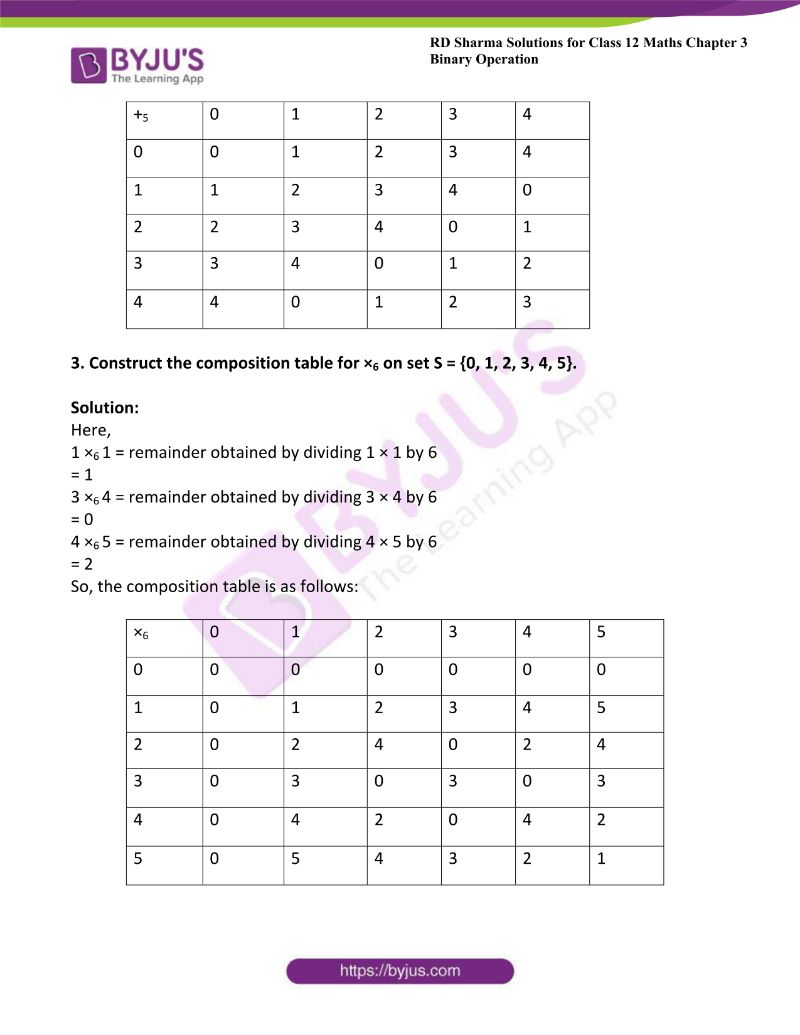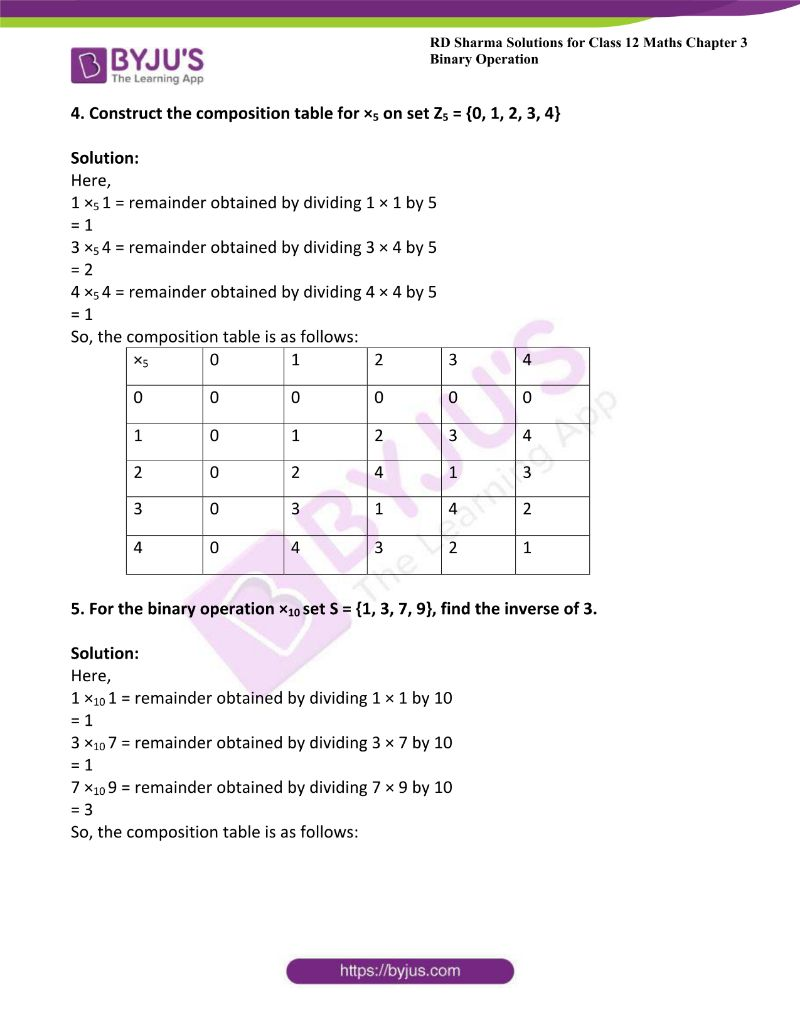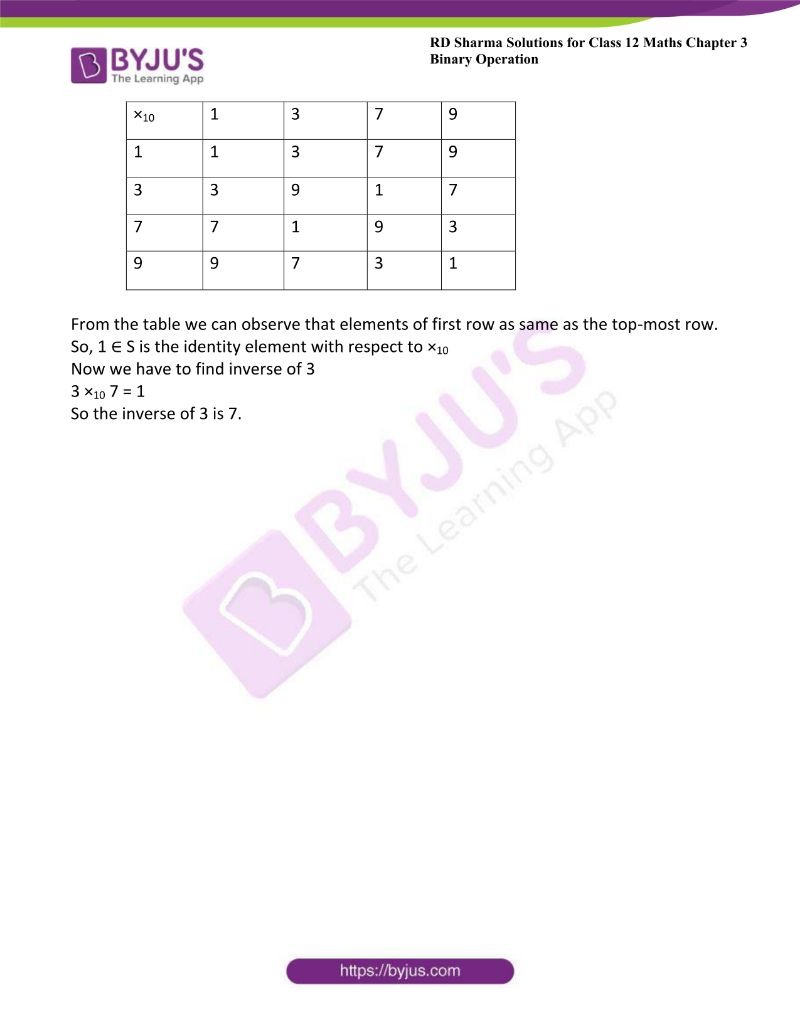### Access RD Sharma Solutions For Class 12 Maths Chapter 3 – Binary Operations

Exercise 3.1 Page No: 3.4

1. Determine whether the following operation define a binary operation on the given set or not:

(i) ‘*’ on N defined by a * b = ab for all a, b ∈ N.

(ii) ‘O’ on Z defined by a O b = ab for all a, b ∈ Z.

(iii)  ‘*’ on N defined by a * b = a + b – 2 for all a, b ∈ N

(iv) ‘×6‘ on S = {1, 2, 3, 4, 5} defined by a ×6 b = Remainder when a b is divided by 6.

(v) ‘+6’ on S = {0, 1, 2, 3, 4, 5} defined by a +6 b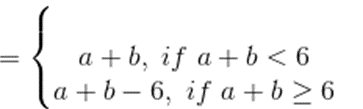(vi) ‘⊙’ on N defined by a ⊙ b= ab + ba for all a, b ∈ N

(vii) ‘*’ on Q defined by a * b = (a – 1)/ (b + 1) for all a, b ∈ Q

Solution:

(i) Given ‘*’ on N defined by a * b = ab for all a, b ∈ N.

Let a, b ∈ N. Then,

a∈ N      [∵ ab≠0 and a, b is positive integer]

⇒ a * b ∈ N

Therefore,

a * b ∈ N, ∀ a, b ∈ N

Thus, * is a binary operation on N.

(ii) Given ‘O’ on Z defined by a O b = ab for all a, b ∈ Z.

Both a = 3 and b = -1 belong to Z.

⇒ a * b = 3-1

= 1/3 ∉ Z

Thus, * is not a binary operation on Z.

(iii)  Given ‘*’ on N defined by a * b = a + b – 2 for all a, b ∈ N

If a = 1 and b = 1,

a * b = a + b – 2

= 1 + 1 – 2

= 0 ∉ N

Thus, there exist a = 1 and b = 1 such that a * b ∉ N

So, * is not a binary operation on N.

(iv) Given ‘×6‘ on S = {1, 2, 3, 4, 5} defined by a ×6 b = Remainder when a b is divided by 6.

Consider the composition table,

 X6 1 2 3 4 5 1 1 2 3 4 5 2 2 4 0 2 4 3 3 0 3 0 3 4 4 2 0 4 2 5 5 4 3 2 1

Here all the elements of the table are not in S.

⇒ For a = 2 and b = 3,

a ×6 b = 2 ×6 3 = remainder when 6 divided by 6 = 0 ≠ S

Thus, ×6 is not a binary operation on S.

(v) Given ‘+6’ on S = {0, 1, 2, 3, 4, 5} defined by a +6 b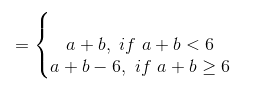Consider the composition table,

 +6 0 1 2 3 4 5 0 0 1 2 3 4 5 1 1 2 3 4 5 0 2 2 3 4 5 0 1 3 3 4 5 0 1 2 4 4 5 0 1 2 3 5 5 0 1 2 3 4

Here all the elements of the table are not in S.

⇒ For a = 2 and b = 3,

a ×6 b = 2 ×6 3 = remainder when 6 divided by 6 = 0 ≠ Thus, ×6 is not a binary operation on S.

(vi) Given ‘⊙’ on N defined by a ⊙ b= ab + ba for all a, b ∈ N

Let a, b ∈ N. Then,

ab, ba ∈ N

⇒ ab + ba ∈ N      [∵Addition is binary operation on N]

⇒ a ⊙ b ∈ N

Thus, ⊙ is a binary operation on N.

(vii) Given ‘*’ on Q defined by a * b = (a – 1)/ (b + 1) for all a, b ∈ Q

If a = 2 and b = -1 in Q,

a * b = (a – 1)/ (b + 1)

= (2 – 1)/ (- 1 + 1)

= 1/0 [which is not defined]

For a = 2 and b = -1

a * b does not belongs to Q

So, * is not a binary operation in Q.

2. Determine whether or not the definition of * given below gives a binary operation. In the event that * is not a binary operation give justification of this.
(i) On Z+, defined * by a * b = a – b

(ii) On Z+, define * by a*b = ab

(iii) On R, define * by a*b = ab2

(iv) On Z+ define * by a * b = |a − b|

(v) On Z+ define * by a * b = a

(vi) On R, define * by a * b = a + 4b2

Here, Z+ denotes the set of all non-negative integers.

Solution:

(i) Given On Z+, defined * by a * b = a – b

If a = 1 and b = 2 in Z+, then

a * b = a – b

= 1 – 2

= -1 ∉ Z+ [because Z+ is the set of non-negative integers]

For a = 1 and b = 2,

a * b ∉ Z+

Thus, * is not a binary operation on Z+.

(ii) Given Z+, define * by a*b = a b

Let a, b ∈ Z+

⇒ a, b ∈ Z+

⇒ a * b ∈ Z+

Thus, * is a binary operation on R.

(iii) Given on R, define by a*b = ab2

Let a, b ∈ R

⇒ a, b2 ∈ R

⇒ ab2 ∈ R

⇒ a * b ∈ R

Thus, * is a binary operation on R.

(iv) Given on Z+ define * by a * b = |a − b|

Let a, b ∈ Z+

⇒ | a – b | ∈ Z+

⇒ a * b ∈ Z+

Therefore,

a * b ∈ Z+, ∀ a, b ∈ Z+

Thus, * is a binary operation on Z+.

(v) Given on Z+ define * by a * b = a

Let a, b ∈ Z+

⇒ a ∈ Z+

⇒ a * b ∈ Z+

Therefore, a * b ∈ Z+ ∀ a, b ∈ Z+

Thus, * is a binary operation on Z+.

(vi) Given On R, define * by a * b = a + 4b2

Let a, b ∈ R

⇒ a, 4b2 ∈ R

⇒ a + 4b2 ∈ R

⇒ a * b ∈ R

Therefore, a *b ∈ R, ∀ a, b ∈ R

Thus, * is a binary operation on R.

3. Let * be a binary operation on the set I of integers, defined by a * b = 2a + b − 3. Find the value of 3 * 4.

Solution:

Given a * b = 2a + b – 3

3 * 4 = 2 (3) + 4 – 3

= 6 + 4 – 3

= 7

4. Is * defined on the set {1, 2, 3, 4, 5} by a * b = LCM of a and b a binary operation? Justify your answer.

Solution:

 LCM 1 2 3 4 5 1 1 2 3 4 5 2 2 2 6 4 10 3 3 5 3 12 15 4 4 4 12 4 20 5 5 10 15 20 5

In the given composition table, all the elements are not in the set {1, 2, 3, 4, 5}.

If we consider a = 2 and b = 3, a * b = LCM of a and b = 6 ∉ {1, 2, 3, 4, 5}.

Thus, * is not a binary operation on {1, 2, 3, 4, 5}.

5. Let S = {a, b, c}. Find the total number of binary operations on S.

Solution:

Number of binary operations on a set with n elements is

$$\begin{array}{l}n^{n^{2}}\end{array}$$

Here, S = {a, b, c}

Number of elements in S = 3

Number of binary operations on a set with 3 elements is

$$\begin{array}{l}3^{3^{2}}\end{array}$$

Exercise 3.2 Page No: 3.12

1. Let ‘*’ be a binary operation on N defined by a * b = l.c.m. (a, b) for all a, b ∈ N
(i) Find 2 * 4, 3 * 5, 1 * 6.

(ii) Check the commutativity and associativity of ‘*’ on N.

Solution:

(i) Given a * b = 1.c.m. (a, b)

2 * 4 = l.c.m. (2, 4)

= 4

3 * 5 = l.c.m. (3, 5)

= 15

1 * 6 = l.c.m. (1, 6)

= 6

(ii) We have to prove commutativity of *

Let a, b ∈ N

a * b = l.c.m (a, b)

= l.c.m (b, a)

= b * a

Therefore

a * b = b * a ∀ a, b ∈ N

Thus * is commutative on N.

Now we have to prove associativity of *

Let a, b, c ∈ N

a * (b * c ) = a * l.c.m. (b, c)

= l.c.m. (a, (b, c))

= l.c.m (a, b, c)

(a * b) * c = l.c.m. (a, b) * c

= l.c.m. ((a, b), c)

= l.c.m. (a, b, c)

Therefore

(a * (b * c) = (a * b) * c, ∀ a, b , c ∈ N

Thus, * is associative on N.

2. Determine which of the following binary operation is associative and which is commutative:

(i) * on N defined by a * b = 1 for all a, b ∈ N

(ii) * on Q defined by a * b = (a + b)/2 for all a, b ∈ Q

Solution:

(i) We have to prove commutativity of *

Let a, b ∈ N

a * b = 1

b * a = 1

Therefore,

a * b = b * a, for all a, b ∈ N

Thus * is commutative on N.

Now we have to prove associativity of *

Let a, b, c ∈ N

Then a * (b * c) = a * (1)

= 1

(a * b) *c = (1) * c

= 1

Therefore a * (b * c) = (a * b) *c for all a, b, c ∈ N

Thus, * is associative on N.

(ii) First we have to prove commutativity of *

Let a, b ∈ N

a * b = (a + b)/2

= (b + a)/2

= b * a

Therefore, a * b = b * a, ∀ a, b ∈ N

Thus * is commutative on N.

Now we have to prove associativity of *

Let a, b, c ∈ N

a * (b * c) = a * (b + c)/2

= [a + (b + c)]/2

= (2a + b + c)/4

Now, (a * b) * c = (a + b)/2 * c

= [(a + b)/2 + c] /2

= (a + b + 2c)/4

Thus, a * (b * c) ≠ (a * b) * c

If a = 1, b= 2, c = 3

1 * (2 * 3) = 1 * (2 + 3)/2

= 1 * (5/2)

= [1 + (5/2)]/2

= 7/4

(1 * 2) * 3 = (1 + 2)/2 * 3

= 3/2 * 3

= [(3/2) + 3]/2

= 4/9

Therefore, there exist a = 1, b = 2, c = 3 ∈ N such that a * (b * c) ≠ (a * b) * c

Thus, * is not associative on N.

3. Let A be any set containing more than one element. Let ‘*’ be a binary operation on A defined by a * b = b for all a, b ∈ A Is ‘*’ commutative or associative on A?

Solution:

Let a, b ∈ A

Then, a * b = b

b * a = a

Therefore a * b ≠ b * a

Thus, * is not commutative on A

Now we have to check associativity:

Let a, b, c ∈ A

a * (b * c) = a * c

= c

Therefore

a * (b * c) = (a * b) * c, ∀ a, b, c ∈ A

Thus, * is associative on A

4. Check the commutativity and associativity of each of the following binary operations:

(i) ‘*’ on Z defined by a * b = a + b + a b for all a, b ∈ Z

(ii) ‘*’ on N defined by a * b = 2ab for all a, b ∈ N

(iii) ‘*’ on Q defined by a * b = a – b for all a, b ∈ Q

(iv) ‘⊙’ on Q defined by a ⊙ b = a2 + b2 for all a, b ∈ Q

(v) ‘o’ on Q defined by a o b = (ab/2) for all a, b ∈ Q

(vi) ‘*’ on Q defined by a * b = ab2 for all a, b ∈ Q

(vii) ‘*’ on Q defined by a * b = a + a b for all a, b ∈ Q

(viii) ‘*’ on R defined by a * b = a + b -7 for all a, b ∈ R

(ix) ‘*’ on Q defined by a * b = (a – b)2 for all a, b ∈ Q

(x) ‘*’ on Q defined by a * b = a b + 1 for all a, b ∈ Q

(xi) ‘*’ on N defined by a * b = ab for all a, b ∈ N

(xii) ‘*’ on Z defined by a * b = a – b for all a, b ∈ Z

(xiii) ‘*’ on Q defined by a * b = (ab/4) for all a, b ∈ Q

(xiv) ‘*’ on Z defined by a * b = a + b – ab for all a, b ∈ Z

(xv) ‘*’ on Q defined by a * b = gcd (a, b) for all a, b ∈ Q

Solution:

(i) First we have to check commutativity of *

Let a, b ∈ Z

Then a * b = a + b + ab

= b + a + ba

= b * a

Therefore,

a * b = b * a, ∀ a, b ∈ Z

Now we have to prove associativity of *

Let a, b, c ∈ Z, Then,

a * (b * c) = a * (b + c + b c)

= a + (b + c + b c) + a (b + c + b c)

= a + b + c + b c + a b + a c + a b c

(a * b) * c = (a + b + a b) * c

= a + b + a b + c + (a + b + a b) c

= a + b + a b + c + a c + b c + a b c

Therefore,

a * (b * c) = (a * b) * c, ∀ a, b, c ∈ Z

Thus, * is associative on Z.

(ii) First we have to check commutativity of *

Let a, b ∈ N

a * b = 2ab

= 2ba

= b * a

Therefore, a * b = b * a, ∀ a, b ∈ N

Thus, * is commutative on N

Now we have to check associativity of *

Let a, b, c ∈ N

Then, a * (b * c) = a * (2bc)

=

$$\begin{array}{l}2^{a*2^{bc}}\end{array}$$

(a * b) * c = (2ab) * c

=

$$\begin{array}{l}2^{ab*2^{c}}\end{array}$$

Therefore, a * (b * c) ≠ (a * b) * c

Thus, * is not associative on N

(iii) First we have to check commutativity of *

Let a, b ∈ Q, then

a * b = a – b

b * a = b – a

Therefore, a * b ≠ b * a

Thus, * is not commutative on Q

Now we have to check associativity of *

Let a, b, c ∈ Q, then

a * (b * c) = a * (b – c)

= a – (b – c)

= a – b + c

(a * b) * c = (a – b) * c

= a – b – c

Therefore,

a * (b * c) ≠ (a * b) * c

Thus, * is not associative on Q

(iv) First we have to check commutativity of ⊙

Let a, b ∈ Q, then

a ⊙ b = a2 + b2

= b2 + a2

= b ⊙ a

Therefore, a ⊙ b = b ⊙ a, ∀ a, b ∈ Q

Thus, ⊙ on Q

Now we have to check associativity of ⊙

Let a, b, c ∈ Q, then

a ⊙ (b ⊙ c) = a ⊙ (b2 + c2)

= a2 + (b2 + c2)2

= a2 + b4 + c4 + 2b2c2

(a ⊙ b) ⊙ c = (a2 + b2) ⊙ c

= (a2 + b2)2 + c2

= a4 + b4 + 2a2b2 + c2

Therefore,

(a ⊙ b) ⊙ c ≠ a ⊙ (b ⊙ c)

Thus, ⊙ is not associative on Q.

(v) First we have to check commutativity of o

Let a, b ∈ Q, then

a o b = (ab/2)

= (b a/2)

= b o a

Therefore, a o b = b o a, ∀ a, b ∈ Q

Thus, o is commutative on Q

Now we have to check associativity of o

Let a, b, c ∈ Q, then

a o (b o c) = a o (b c/2)

= [a (b c/2)]/2

= [a (b c/2)]/2

= (a b c)/4

(a o b) o c = (ab/2) o c

= [(ab/2) c] /2

= (a b c)/4

Therefore a o (b o c) = (a o b) o c, ∀ a, b, c ∈ Q

Thus, o is associative on Q.

(vi) First we have to check commutativity of *

Let a, b ∈ Q, then

a * b = ab2

b * a = ba2

Therefore,

a * b ≠ b * a

Thus, * is not commutative on Q

Now we have to check associativity of *

Let a, b, c ∈ Q, then

a * (b * c) = a * (bc2)

= a (bc2)2

= ab2 c4

(a * b) * c = (ab2) * c

= ab2c2

Therefore a * (b * c) ≠ (a * b) * c

Thus, * is not associative on Q.

(vii) First we have to check commutativity of *

Let a, b ∈ Q, then

a * b = a + ab

b * a = b + ba

= b + ab

Therefore, a * b ≠ b * a

Thus, * is not commutative on Q.

Now we have to prove associativity on Q.

Let a, b, c ∈ Q, then

a * (b * c) = a * (b + b c)

= a + a (b + b c)

= a + ab + a b c

(a * b) * c = (a + a b) * c

= (a + a b) + (a + a b) c

= a + a b + a c + a b c

Therefore a * (b * c) ≠ (a * b) * c

Thus, * is not associative on Q.

(viii) First we have to check commutativity of *

Let a, b ∈ R, then

a * b = a + b – 7

= b + a – 7

= b * a

Therefore,

a * b = b * a, for all a, b ∈ R

Thus, * is commutative on R

Now we have to prove associativity of * on R.

Let a, b, c ∈ R, then

a * (b * c) = a * (b + c – 7)

= a + b + c -7 -7

= a + b + c – 14

(a * b) * c = (a + b – 7) * c

= a + b – 7 + c – 7

= a + b + c – 14

Therefore,

a * (b * c ) = (a * b) * c, for all a, b, c ∈ R

Thus, * is associative on R.

(ix) First we have to check commutativity of *

Let a, b ∈ Q, then

a * b = (a – b)2

= (b – a)2

= b * a

Therefore,

a * b = b * a, for all a, b ∈ Q

Thus, * is commutative on Q

Now we have to prove associativity of * on Q

Let a, b, c ∈ Q, then

a * (b * c) = a * (b – c)2

= a * (b2 + c2 – 2 b c)

= (a – b2 – c2 + 2bc)2

(a * b) * c = (a – b)2 * c

= (a2 + b2 – 2ab) * c

= (a2 + b2 – 2ab – c)2

Therefore, a * (b * c) ≠ (a * b) * c

Thus, * is not associative on Q.

(x) First we have to check commutativity of *

Let a, b ∈ Q, then

a * b = ab + 1

= ba + 1

= b * a

Therefore

a * b = b * a, for all a, b ∈ Q

Thus, * is commutative on Q

Now we have to prove associativity of * on Q

Let a, b, c ∈ Q, then

a * (b * c) = a * (bc + 1)

= a (b c + 1) + 1

= a b c + a + 1

(a * b) * c = (ab + 1) * c

= (ab + 1) c + 1

= a b c + c + 1

Therefore, a * (b * c) ≠ (a * b) * c

Thus, * is not associative on Q.

(xi) First we have to check commutativity of *

Let a, b ∈ N, then

a * b = ab

b * a = ba

Therefore, a * b ≠ b * a

Thus, * is not commutative on N.

Now we have to check associativity of *

a * (b * c) = a * (bc)

=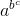(a * b) * c = (ab) * c

= (ab)c

= abc

Therefore, a * (b * c) ≠ (a * b) * c

Thus, * is not associative on N

(xii) First we have to check commutativity of *

Let a, b ∈ Z, then

a * b = a – b

b * a = b – a

Therefore,

a * b ≠ b * a

Thus, * is not commutative on Z.

Now we have to check associativity of *

Let a, b, c ∈ Z, then

a * (b * c) = a * (b – c)

= a – (b – c)

= a – (b + c)

(a * b) * c = (a – b) – c

= a – b – c

Therefore, a * (b * c) ≠ (a * b) * c

Thus, * is not associative on Z

(xiii) First we have to check commutativity of *

Let a, b ∈ Q, then

a * b = (ab/4)

= (ba/4)

= b * a

Therefore, a * b = b * a, for all a, b ∈ Q

Thus, * is commutative on Q

Now we have to check associativity of *

Let a, b, c ∈ Q, then

a * (b * c) = a * (b c/4)

= [a (b c/4)]/4

= (a b c/16)

(a * b) * c = (ab/4) * c

= [(ab/4) c]/4

= a b c/16

Therefore,

a * (b * c) = (a * b) * c for all a, b, c ∈ Q

Thus, * is associative on Q.

(xiv) First we have to check commutativity of *

Let a, b ∈ Z, then

a * b = a + b – ab

= b + a – ba

= b * a

Therefore, a * b = b * a, for all a, b ∈ Z

Thus, * is commutative on Z.

Now we have to check associativity of *

Let a, b, c ∈ Z

a * (b * c) = a * (b + c – b c)

= a + b + c- b c – ab – ac + a b c

(a * b) * c = (a + b – a b) c

= a + b – ab + c – (a + b – ab) c

= a + b + c – ab – ac – bc + a b c

Therefore,

a * (b * c) = (a * b) * c, for all a, b, c ∈ Z

Thus, * is associative on Z.

(xv) First we have to check commutativity of *

Let a, b ∈ N, then

a * b = gcd (a, b)

= gcd (b, a)

= b * a

Therefore, a * b = b * a, for all a, b ∈ N

Thus, * is commutative on N.

Now we have to check associativity of *

Let a, b, c ∈ N

a * (b * c) = a * [gcd (a, b)]

= gcd (a, b, c)

(a * b) * c = [gcd (a, b)] * c

= gcd (a, b, c)

Therefore,

a * (b * c) = (a * b) * c, for all a, b, c ∈ N

Thus, * is associative on N.

5. If the binary operation o is defined by a0b = a + b – ab on the set Q – {-1} of all rational numbers other than 1, show that o is commutative on Q – .

Solution:

Let a, b ∈ Q – {-1}.

Then aob = a + b – ab

= b+ a – ba

= boa

Therefore,

aob = boa for all a, b ∈ Q – {-1}

Thus, o is commutative on Q – {-1}

6. Show that the binary operation * on Z defined by a * b = 3a + 7b is not commutative?

Solution:

Let a, b ∈ Z

a * b = 3a + 7b

b * a = 3b + 7a

Thus, a * b ≠ b * a

Let a = 1 and b = 2

1 * 2 = 3 × 1 + 7 × 2

= 3 + 14

= 17

2 * 1 = 3 × 2 + 7 × 1

= 6 + 7

= 13

Therefore, there exist a = 1, b = 2 ∈ Z such that a * b ≠ b * a

Thus, * is not commutative on Z.

7. On the set Z of integers a binary operation * is defined by a 8 b = ab + 1 for all a, b ∈ Z. Prove that * is not associative on Z.

Solution:

Let a, b, c ∈ Z

a * (b * c) = a * (bc + 1)

= a (bc + 1) + 1

= a b c + a + 1

(a * b) * c = (ab+ 1) * c

= (ab + 1) c + 1

= a b c + c + 1

Thus, a * (b * c) ≠ (a * b) * c

Thus, * is not associative on Z.

Exercise 3.3 Page No: 3.15

1. Find the identity element in the set I+ of all positive integers defined by a * b = a + b for all a, b ∈ I+.

Solution:

Let e be the identity element in I+ with respect to * such that

a * e = a = e * a, ∀ a ∈ I+

a * e = a and e * a = a, ∀ a ∈ I+

a + e = a and e + a = a, ∀ a ∈ I+

e = 0, ∀ a ∈ I+

Thus, 0 is the identity element in I+ with respect to *.

2. Find the identity element in the set of all rational numbers except – 1 with respect to * defined by a * b = a + b + ab

Solution:

Let e be the identity element in I+ with respect to * such that

a * e = a = e * a, ∀ a ∈ Q – {-1}

a * e = a and e * a = a, ∀ a ∈ Q – {-1}

a + e + ae = a and e + a + ea = a, ∀ a ∈ Q – {-1}

e + ae = 0 and e + ea = 0, ∀ a ∈ Q – {-1}

e (1 + a) = 0 and e (1 + a) = 0, ∀ a ∈ Q – {-1}

e = 0, ∀ a ∈ Q – {-1} [because a not equal to -1]

Thus, 0 is the identity element in Q – {-1} with respect to *.

Exercise 3.4 Page No: 3.25

1. Let * be a binary operation on Z defined by a * b = a + b – 4 for all a, b ∈ Z.

(i) Show that * is both commutative and associative.

(ii) Find the identity element in Z

(iii) Find the invertible element in Z.

Solution:

(i) First we have to prove commutativity of *

Let a, b ∈ Z. then,

a * b = a + b – 4

= b + a – 4

= b * a

Therefore,

a * b = b * a, ∀ a, b ∈ Z

Thus, * is commutative on Z.

Now we have to prove associativity of Z.

Let a, b, c ∈ Z. then,

a * (b * c) = a * (b + c – 4)

= a + b + c -4 – 4

= a + b + c – 8

(a * b) * c = (a + b – 4) * c

= a + b – 4 + c – 4

= a + b + c – 8

Therefore,

a * (b * c) = (a * b) * c, for all a, b, c ∈ Z

Thus, * is associative on Z.

(ii) Let e be the identity element in Z with respect to * such that

a * e = a = e * a ∀ a ∈ Z

a * e = a and e * a = a, ∀ a ∈ Z

a + e – 4 = a and e + a – 4 = a, ∀ a ∈ Z

e = 4, ∀ a ∈ Z

Thus, 4 is the identity element in Z with respect to *.

(iii) Let a ∈ Z and b ∈ Z be the inverse of a. Then,

a * b = e = b * a

a * b = e and b * a = e

a + b – 4 = 4 and b + a – 4 = 4

b = 8 – a ∈ Z

Thus, 8 – a is the inverse of a ∈ Z

2. Let * be a binary operation on Q0 (set of non-zero rational numbers) defined by a * b = (3ab/5) for all a, b ∈ Q0. Show that * is commutative as well as associative. Also, find its identity element, if it exists.

Solution:

First we have to prove commutativity of *

Let a, b ∈ Q0

a * b = (3ab/5)

= (3ba/5)

= b * a

Therefore, a * b = b * a, for all a, b ∈ Q0

Now we have to prove associativity of *

Let a, b, c ∈ Q0

a * (b * c) = a * (3bc/5)

= [a (3 bc/5)] /5

= 3 abc/25

(a * b) * c = (3 ab/5) * c

= [(3 ab/5) c]/ 5

= 3 abc /25

Therefore a * (b * c) = (a * b) * c, for all a, b, c ∈ Q0

Thus * is associative on Q0

Now we have to find the identity element

Let e be the identity element in Z with respect to * such that

a * e = a = e * a ∀ a ∈ Q0

a * e = a and e * a = a, ∀ a ∈ Q0

3ae/5 = a and 3ea/5 = a, ∀ a ∈ Q0

e = 5/3 ∀ a ∈ Q0 [because a is not equal to 0]

Thus, 5/3 is the identity element in Q0 with respect to *.

3. Let * be a binary operation on Q – {-1} defined by a * b = a + b + ab for all a, b ∈ Q – {-1}. Then,

(i) Show that * is both commutative and associative on Q – {-1}

(ii) Find the identity element in Q – {-1}

(iii) Show that every element of Q – {-1} is invertible. Also, find inverse of an arbitrary element.

Solution:

(i) First we have to check commutativity of *

Let a, b ∈ Q – {-1}

Then a * b = a + b + ab

= b + a + ba

= b * a

Therefore,

a * b = b * a, ∀ a, b ∈ Q – {-1}

Now we have to prove associativity of *

Let a, b, c ∈ Q – {-1}, Then,

a * (b * c) = a * (b + c + b c)

= a + (b + c + b c) + a (b + c + b c)

= a + b + c + b c + a b + a c + a b c

(a * b) * c = (a + b + a b) * c

= a + b + a b + c + (a + b + a b) c

= a + b + a b + c + a c + b c + a b c

Therefore,

a * (b * c) = (a * b) * c, ∀ a, b, c ∈ Q – {-1}

Thus, * is associative on Q – {-1}.

(ii) Let e be the identity element in I+ with respect to * such that

a * e = a = e * a, ∀ a ∈ Q – {-1}

a * e = a and e * a = a, ∀ a ∈ Q – {-1}

a + e + ae = a and e + a + ea = a, ∀ a ∈ Q – {-1}

e + ae = 0 and e + ea = 0, ∀ a ∈ Q – {-1}

e (1 + a) = 0 and e (1 + a) = 0, ∀ a ∈ Q – {-1}

e = 0, ∀ a ∈ Q – {-1} [because a not equal to -1]

Thus, 0 is the identity element in Q – {-1} with respect to *.

(iii) Let a ∈ Q – {-1} and b ∈ Q – {-1} be the inverse of a. Then,

a * b = e = b * a

a * b = e and b * a = e

a + b + ab = 0 and b + a + ba = 0

b (1 + a) = – a Q – {-1}

b = -a/1 + a Q – {-1} [because a not equal to -1]

Thus, -a/1 + a is the inverse of a ∈ Q – {-1}

4. Let A = R0 × R, where R0 denote the set of all non-zero real numbers. A binary operation ‘O’ is defined on A as follows: (a, b) O (c, d) = (ac, bc + d) for all (a, b), (c, d) ∈ R0 × R.

(i) Show that ‘O’ is commutative and associative on A

(ii) Find the identity element in A

(iii) Find the invertible element in A.

Solution:

(i) Let X = (a, b) and Y = (c, d) ∈ A, ∀ a, c ∈ R0 and b, d ∈ R

Then, X O Y = (ac, bc + d)

And Y O X = (ca, da + b)

Therefore,

X O Y = Y O X, ∀ X, Y ∈ A

Thus, O is not commutative on A.

Now we have to check associativity of O

Let X = (a, b), Y = (c, d) and Z = (e, f), ∀ a, c, e ∈ R0 and b, d, f ∈ R

X O (Y O Z) = (a, b) O (ce, de + f)

= (ace, bce + de + f)

(X O Y) O Z = (ac, bc + d) O (e, f)

= (ace, (bc + d) e + f)

= (ace, bce + de + f)

Therefore, X O (Y O Z) = (X O Y) O Z, ∀ X, Y, Z ∈ A

(ii) Let E = (x, y) be the identity element in A with respect to O, ∀ x ∈ R0 and y ∈ R

Such that,

X O E = X = E O X, ∀ X ∈ A

X O E = X and EOX = X

(ax, bx +y) = (a, b) and (xa, ya + b) = (a, b)

Considering (ax, bx + y) = (a, b)

ax = a

x = 1

And bx + y = b

y = 0 [since x = 1]

Considering (xa, ya + b) = (a, b)

xa = a

x = 1

And ya + b = b

y = 0 [since x = 1]

Therefore (1, 0) is the identity element in A with respect to O.

(iii) Let F = (m, n) be the inverse in A ∀ m ∈ R0 and n ∈ R

X O F = E and F O X = E

(am, bm + n) = (1, 0) and (ma, na + b) = (1, 0)

Considering (am, bm + n) = (1, 0)

am = 1

m = 1/a

And bm + n = 0

n = -b/a [since m = 1/a]

Considering (ma, na + b) = (1, 0)

ma = 1

m = 1/a

And na + b = 0

n = -b/a

Therefore the inverse of (a, b) ∈ A with respect to O is (1/a, -b/a)

Exercise 3.5 Page No: 3.33

1. Construct the composition table for ×4 on set S = {0, 1, 2, 3}.

Solution:

Given that ×4 on set S = {0, 1, 2, 3}

Here,

1 ×4 1 = remainder obtained by dividing 1 × 1 by 4

= 1

0 ×4 1 = remainder obtained by dividing 0 × 1 by 4

= 0

2 ×4 3 = remainder obtained by dividing 2 × 3 by 4

= 2

3 ×4 3 = remainder obtained by dividing 3 × 3 by 4

= 1

So, the composition table is as follows:

 ×4 0 1 2 3 0 0 0 0 0 1 0 1 2 3 2 0 2 0 2 3 0 3 2 1

2. Construct the composition table for +5 on set S = {0, 1, 2, 3, 4}

Solution:

1 +5 1 = remainder obtained by dividing 1 + 1 by 5

= 2

3 +5 1 = remainder obtained by dividing 3 + 1 by 5

= 2

4 +5 1 = remainder obtained by dividing 4 + 1 by 5

= 3

So, the composition table is as follows:

 +5 0 1 2 3 4 0 0 1 2 3 4 1 1 2 3 4 0 2 2 3 4 0 1 3 3 4 0 1 2 4 4 0 1 2 3

3. Construct the composition table for ×6 on set S = {0, 1, 2, 3, 4, 5}.

Solution:

Here,

1 ×6 1 = remainder obtained by dividing 1 × 1 by 6

= 1

3 ×6 4 = remainder obtained by dividing 3 × 4 by 6

= 0

4 ×6 5 = remainder obtained by dividing 4 × 5 by 6

= 2

So, the composition table is as follows:

 ×6 0 1 2 3 4 5 0 0 0 0 0 0 0 1 0 1 2 3 4 5 2 0 2 4 0 2 4 3 0 3 0 3 0 3 4 0 4 2 0 4 2 5 0 5 4 3 2 1

4. Construct the composition table for ×5 on set Z5 = {0, 1, 2, 3, 4}

Solution:

Here,

1 ×5 1 = remainder obtained by dividing 1 × 1 by 5

= 1

3 ×5 4 = remainder obtained by dividing 3 × 4 by 5

= 2

4 ×5 4 = remainder obtained by dividing 4 × 4 by 5

= 1

So, the composition table is as follows:

 ×5 0 1 2 3 4 0 0 0 0 0 0 1 0 1 2 3 4 2 0 2 4 1 3 3 0 3 1 4 2 4 0 4 3 2 1

5. For the binary operation ×10 set S = {1, 3, 7, 9}, find the inverse of 3.

Solution:

Here,

1 ×10 1 = remainder obtained by dividing 1 × 1 by 10

= 1

3 ×10 7 = remainder obtained by dividing 3 × 7 by 10

= 1

7 ×10 9 = remainder obtained by dividing 7 × 9 by 10

= 3

So, the composition table is as follows:

 ×10 1 3 7 9 1 1 3 7 9 3 3 9 1 7 7 7 1 9 3 9 9 7 3 1

From the table we can observe that elements of first row as same as the top-most row.

So, 1 ∈ S is the identity element with respect to ×10

Now we have to find inverse of 3

3 ×10 7 = 1

So the inverse of 3 is 7.

### Also, Access RD Sharma Solutions for Class 12 Maths Chapter 3 Binary Operations

Exercise 3.1 Solutions

Exercise 3.2 Solutions

Exercise 3.3 Solutions

Exercise 3.4 Solutions

Exercise 3.5 Solutions

## RD Sharma Class 12 Solutions Chapter 3 Binary Operations

Let us have a look at some of the important concepts that are discussed in this chapter.

• Definition and meaning of binary operations
• Number of binary operations
• Types of binary operations
• Commutativity
• Associativity
• Distributivity
• Identity element
• The inverse of an element
• Composition table
• Multiplication modulo

## Frequently Asked Questions on RD Sharma Solutions for Class 12 Chapter 3

### Where can I download the RD Sharma Solutions for Class 12 Maths Chapter 3?

The RD Sharma Solutions for Class 12 Maths Chapter 3 are available in both online and offline mode from BYJU’S website. You can easily download the PDF version of the RD Sharma Class 12 Solutions for free. Subjects experts at BYJU’S have prepared the RD Sharma Solutions in accordance with the latest CBSE guidelines and marking schemes.

### Where can students obtain the correct RD Sharma Class 12 Solutions Chapter 3?

Students can obtain the accurate RD Sharma Class 12 Solutions directly from BYJU’S website. RD Sharma textbooks are the best study materials providing both students and teachers with a wide range of practice questions. RD Sharma Solutions are prepared with utmost care to help students understand concepts easily and ace their board exams.

### Is RD Sharma Solutions Class 12 Maths Chapter 3 the best study material for exam preparation?

Yes, the RD Sharma Solutions Class 12 Maths is the best study material for CBSE board exam preparation. These solutions are curated by subject experts at BYJU’S outlining the main concepts clearly and precisely. For students aspiring to obtain an excellent score in the Class 12 Maths board exam, referring to RD Sharma Solutions is a must.

### Why should I choose RD Sharma Solutions for Class 12 Maths Chapter 3?

The RD Sharma Solutions framed by experts at BYJU’S provides numerous benefits for the students preparing for their final exam. Each concept offers a detailed explanation and helps students to score good marks. The solutions strictly adhere to the current syllabus of the CBSE 2021-22. Apart from Class 12 board exams, these solutions will also help students to prepare for other competitive exams as well.

### What are the concepts covered in RD Sharma Solutions for Class 12 Maths Chapter 3?

The concepts covered in RD Sharma Solutions for Class 12 Maths Chapter 3 are as follows:
• Definition and meaning of binary operations
• Number of binary operations
• Types of binary operations
• Commutativity
• Associativity
• Distributivity
• Identity element
• The inverse of an element
• Composition table
• Multiplication modulo